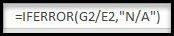top of page

# Getting Rid of the #DIV/0! Error in Excel

Do you ever receive the below pesky error when you are creating calculations in Excel?This error occurs when you are trying to divide by zero. There is an easy way to remedy this error. Simply add an IFERROR formula to your calculation.Change the formula from something like =G2/F2 to =IFERROR(G2/E2,0)

Your calculation is the first item after the open parenthesis. Anything after the comma will be the metric that will replace the error. In this case, the formula will replace a zero if it runs into an error. You could also enter text if you wanted to instead of a zero such as below;